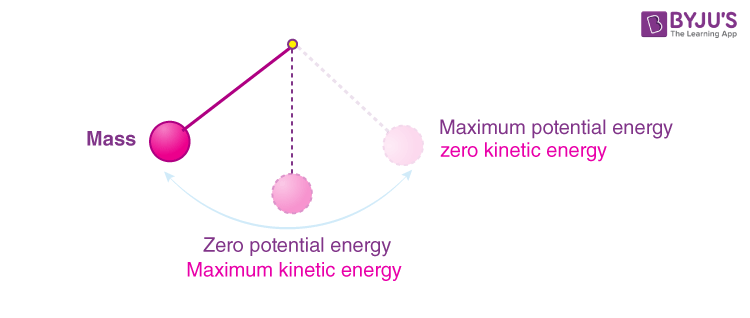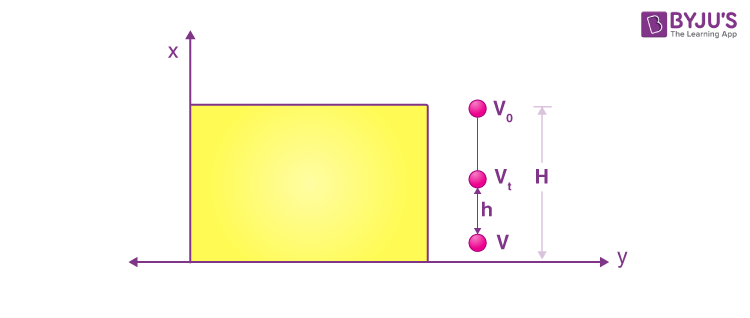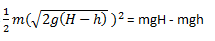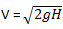# Conservation of Mechanical Energy

The capacity to do work is known as energy. We have heard of many types of energy like mechanical energy, potential energy, chemical energy, kinetic energy, thermal energy, solar energy, and many more. In this article, let us discuss in detail the conservation of mechanical energy.

## What is Mechanical Energy?

Mechanical energy is the sum of kinetic energy and potential energy in an object that is used to do a particular work. In other words, it describes the energy of an object because of its motion or position, or both.

Let us consider the example of an ideal simple pendulum (friction-less). We can see that the mechanical energy of this system is a combination of its kinetic energy and gravitational potential energy. As the pendulum swings back and forth, a constant exchange between the kinetic energy and potential energy takes place. When the bob attains its maximum height, the potential energy of the system is the highest, whereas the kinetic energy is zero. At the mean position, the kinetic energy is the highest, and the potential energy is zero. Between these two extreme points, we see that the system possesses both kinetic and potential energy, the sum of which is constant. These observations tell us a lot about the conservation of mechanical energy. But how can we prove it for every other system? In the next section, we shall learn more about the conservation of mechanical energy using a suitable example.## Conservation of Mechanical Energy

According to the principle of conservation of mechanical energy,

The total mechanical energy of a system is conserved i.e., the energy can neither be created nor be destroyed; it can only be internally converted from one form to another if the forces doing work on the system are conservative in nature.

In order to understand this statement more clearly, let us consider an example of one-dimensional motion of a system. Here a body, under the action of a conservative force F, gets displaced by Δx, then from the work-energy theorem, we can say that the network done by all the forces acting on a system is equal to the change in the kinetic energy of the system.

Mathematically, ΔKE = F(x) Δx

Where, ΔK is the change in kinetic energy of the system. Considering only conservative forces are acting on the system Wnet = Wc.

Thus Wc = ΔKE

Also, If conservative forces do the work in a system, the system loses potential energy equal to the work done. Hence, Wc = -PE.

Which implies that the total kinetic energy and potential energy of a system remains constant if the process involves only conservative forces.

KE + PE = constant

KEi+ PEi = KEf+ PEf

Where denotes the initial values and f denotes the final values of KE and PE.

This law applies only to the extent that the forces are conservative in nature. The mechanical energy of the system is defined as the total kinetic energy plus the total potential energy. In a system that comprises only conservative forces, each force is associated with a form of potential energy and the energy only changes between the kinetic energy and different types of potential energy, such that the total energy remains constant.

Following is the table explaining other related concepts:

## Example To Explain Total Mechanical Energy Of The System

Example 1Let us understand this principle more clearly with the following example. Let us say, a ball of mass m is dropped from a cliff of height H, as shown above.

At height H:

Potential energy (PE) = m×g×H

Kinetic energy (K.E.) = 0

Total mechanical energy = mgH

At height h:

Potential energy(PE) = m×g×h

Kinetic energy (K.E.) =1/2(mv^2)

Using the equations of motion, the velocity v1 at a height h for an object of mass m falling from a height H can be written asHence, the kinetic energy can be given as,Total mechanical energy = (mgH – mgh) – mgh = mgH

At height zero:

Potential energy: 0

Kinetic energy: 1/2(mv^2)

Using the equations of motion we can see that velocity v at the bottom of the cliff, just before touching the ground isHence, the kinetic energy can be given as,Total mechanical energy: mgH

We saw the total mechanical energy of the system is constant throughout.
To know about kinetic energy and potential energy in detail, see the video below.## Frequently Asked Questions – FAQs

Q1

### State the principle of conservation of mechanical energy.

The total mechanical energy of a system is conserved i.e., the energy can neither be created nor be destroyed; it can only be internally converted from one form to another if the forces doing work on the system are conservative in nature.
Q2

### Define mechanical energy of the system

The mechanical energy of the system is defined as the total kinetic energy plus the total potential energy.

Q3

### Is kinetic energy conserved in elastic collisions?

Yes, kinetic energy is conserved in elastic collisions.

Q4

Electric motor.
Q5

Heat engine.